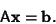## Gaussian Elimination

A method for solving Matrix Equations of the form(1)

Starting with the system of equations(2)

compose the augmented Matrix equation''(3)

Then, perform Matrix operations to put the augmented Matrix into the form(4)

Solve the equation of theth row for, then substitute back into the equation of thest row obtain a solution for, etc., according to the formula(5)